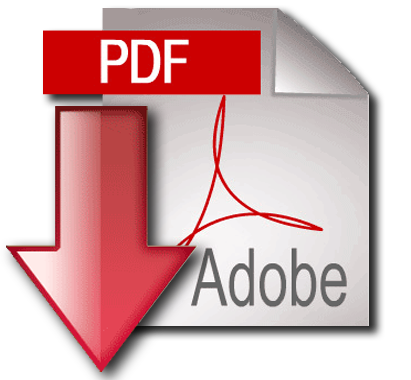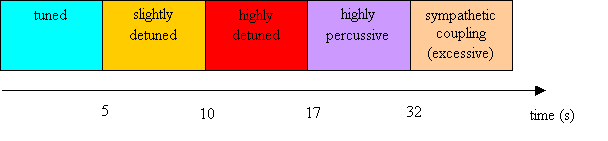## Analysis and synthesis of piano sounds using physical and signal modelsAuthors: Bensa J.
Publication Date: May 2003 (PhD thesis, Aix-Marseille University, 2003)

Tags:

Source-resonator approach (see section 2.5.1)

1. Note B0, recorded in an anechoic room using an artificial head, followed by the note B0 again, after removing the first 20 ms of the signal. Are the two signals perceptually identical?

2.  A7, recorded in an anechoic room using an artificial head, followed by the note A7 again, after removing the first 10 ms of the signal. Are the two signals perceptually identical?

Analysis and resynthesis (in the frequency domain) of the vibration of single or multiple struck strings using single or coupled digital waveguides respectively (see section 2.5). The original signals are measured on the experimental setup (see section 2.6.2), using an accelerometer at the bridge.

3. Original signal, one string (figure 2.23)

4. Resynthesized signal, one string (figure 2.23)

5. Original signal, two strings (figure 2.24)

6. Resynthesized signal, two strings (figure 2.24)

7. Original signal, three strings (figure 2.25)

8. Resynthesized signal, three strings (figure 2.25)

Parameter optimization (see section 3.5)

9. Original signal, velocity, recorded on the experimental setup, using a laser vibrometer (figures 3.4 and 3.5)

10. Synthesized signal, using finite differences and the parameters obtained by measurements or found in the literature

11. Synthesized signal, using finite differences and the parameters obtained after optimization (figures 3.4 and 3.5)

Digital wave filters and digital waveguides (see section 3.7)

12. Synthesized sound obtained by connecting the wave digital piano hammer to a digital waveguide (figure 3.11)

Analysis and resynthesis (in the frequency domain) of the vibration of a single struck piano string using one digital waveguide. The original sound (velocity) is measured on a piano (see section 4.3) using an accelerometer at the bridge. The resonator is estimated using the methods described in section 2.6.

13. Original sound, note E1

14. Impulse response of the resonator

15. Excitation obtained by deconvolution

16. Resynthesized sound by convolution between the excitation and the impulse response of the resonator

Analysis and resynthesis (in the frequency domain) of a piano piece. “Fantaisie” from K.P. Bach (see section 4.3)

17. Original signal, acceleration, recorded on a Yamaha Disklavier, at one point of the bridge using an accelerometer. All the notes are played at the same velocity.

18. Reynthesized signal, using simple and coupled digital waveguides. The resonator are estimated using the methods described in section 2.6. The excitations used are those obtained by deconvolution. The signal corresponding to each note has been computed in the frequency domain and the whole score has been obtained by mixing. The energy transfer between strings of different notes is then not taken into account.

Excitation model (see section 3.8 and 4.4)

19. Note G2, recorded in an anechoic room using an artificial head, played pianissimo and fortissimo. The two signals have been normalized.

20. Synthesized excitation signal, for 14 hammer velocities (see section 4.4)

Analysis and resynthesis using the “full model” in the frequency domain (the resonator model and the excitation model, see section 4). The original signals (velocities) are recorded on a Yamaha Disklavier, on the bridge using an accelerometer.

21. Note C2, original sound

22. Note C2, synthesized sound, two coupled waveguides

23. Note C3, original sound

24. Note C3, synthesized sound, three coupled waveguides

Synthesis in the frequency domain using the resonator interface (using analytic modulus and phases see equations 2.27a and 2.27b and section 5.2.1)

25.Synthesized sound, note E1, using the “regular” parameters

26. Synthesized sound, note E1 for a highly stiff string

27. Synthesized sound, note C2, using “regular” parameters

28. Synthesized sound, note C2. The first string is highly stiff and highly damped, the second is “regular”.

29. Synthesized sound, note B2 using “regular” parameters

30. Synthesized sound, note B2. The three strings are highly detuned.

Synthesis in the time domain (see sections 5.3 and 5.4)

31. Sequence played using the real-time synthesizer and recorded. Some parameters are modified in real-time.